JEE  >  Irodov Solutions: Electromagnetic Waves. Radiation- 2

# Irodov Solutions: Electromagnetic Waves. Radiation- 2 - Notes | Study I. E. Irodov Solutions for Physics Class 11 & Class 12 - JEE

 1 Crore+ students have signed up on EduRev. Have you?

Q.211. Demonstrate that a closed system of charged non-relativistic particles with identical specific charges emits no dipole radiation.

Ans.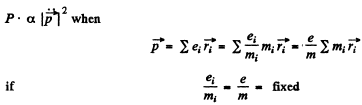But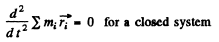Hence         P = 0

Q.212. Find the mean radiation power of an electron performing harmonic oscillations with amplitude a = 0.10 nm and frequen cy  ω = 6.5.1014S-1

Ans.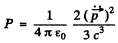Thus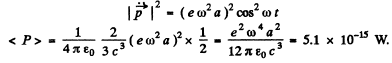Q.213. Find the radiation power developed by a non-relativistic particle with charge e and mass m, moving along a circular orbit of radius R in the field of a stationary point charge q.

Ans. Here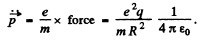Thus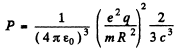Q.214. A particle with charge e and mass m. flies with non-relativistic velocity v at a distance b past a stationary particle with charge q. Neglecting the bending of the trajectory of the moving particle, find the energy lost by this particle due to radiation during the total flight time

Ans. Most of the radiation occurs when the moving particle is closest to the stationary particle. In that region, we can write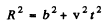and apply the previous problem’s formula

Thus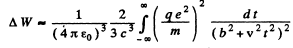(the integral can be taken between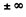with little error.)

Now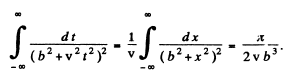Hence,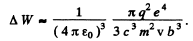Q.215. A non-relativistic proton enters a half-space along the normal to the transverse uniform magnetic field whose induction equals B = 1.0 T. Find the ratio of the energy lost by the proton due to radiation during its motion in the field to its initial kinetic energy.

Ans. For the semicircular path on the right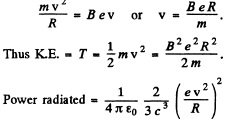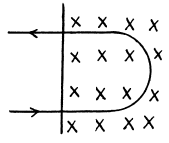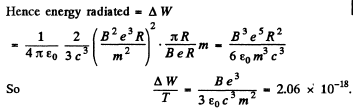(neglecting the change in v due to radiation, correct if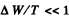).

Q.216. A non-relativistic charged particle moves in a transverse uniform magnetic field with induction B. Find the time dependence of the particle's kinetic energy diminishing due to radiation. How soon will its kinetic energy decrease e-fold? Calculate this time interval for the case
(a) of an electron,
(b) of a proton.

Ans.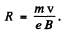Then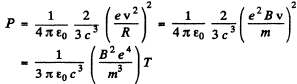This is the radiated power so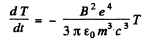Integrating,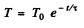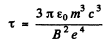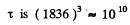times less for an electron than for a proton so electrons radiate away their energy much faster in a magnetic field.

Q.217. A charged particle moves along the y axis according to the law y = a cos ωt, and the point of observation P is located on the axis at a distance 1 from the particle (l ≫ a). Find the ratio of electromagnetic radiation flow densities S1/S2  at the point P at the moments when the coordinate of the particle y1 = 0 and y = a. Calculate that ratio if co = 3.3.106  s--1  and l = 190 m.

Ans. P is a fixed point at a distance l from the equilibrium position of the particle. Because / > a, to first order in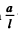the distance between P and the instantaneous position of the particle is still l For the first case y = 0 so t = T/4

The corresponding retarded time is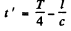Now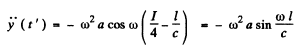For the second case y = a at t = 0 so at the retarded time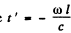Thus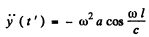The radiation fluxes in the two cases are proportional to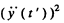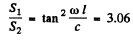on substitution.

Note : The radiation received at P at time t depends on the acceleration of the charge at the retarded time.

Q.218. A charged particle moves uniformly with velocity v along a circle of radius R in the plane xy (Fig. 4.40). An observer is located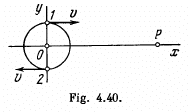on the x axis at a point P which is removed from the centre of the circle by a distance much exceeding R. Find:
(a) the relationship between the observed values of the y projection of the particle's acceleration and the y coordinate of the particle;
(b) the ratio of electromagnetic radiation flow densities S1/Sat the point P at the moments of time when the particle moves, from the standpoint of the observer P, toward him and away from him, as shown in the figure.

Ans. Along the circle x = sin ωt, y = cos ωt

where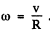If t is the parameter in x (r), y (r) and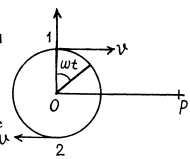t ' is the observer time then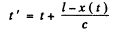where we have neglected the effect of the y--cordinate which is of second order. The observed cordinate are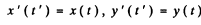Then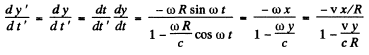and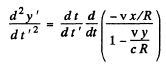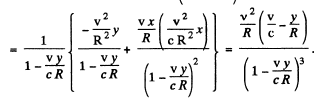This is the observed acceleration.

(b) Energy flow density of JEM radiation S is proportional to the square of the y-projection o f the observed acceleration o f the particle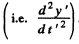Thus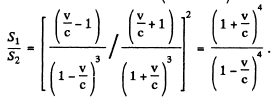Q.219. An electromagnetic wave emitted by an elementary dipole propagates in vacuum so that in the far field zone the mean value of the energy flow density is equal to So  at the point removed from the dipole by a distance r along the perpendicular drawn to the dipole's axis. Find the mean radiation power of the dipole. pole's axis. Find the mean radiation power of the dipole.

Ans. We know that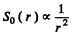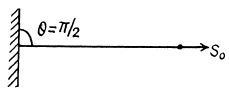At other angles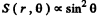Thus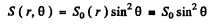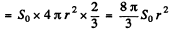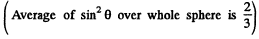Q.220. The mean power radiated by an elementary dipole is equal to Po. Find the mean space density of energy of the electromagnetic field in vacuum in the far field zone at the point removed from the dipole by a distance r along the perpendicular drawn to the dipole's axis.

Ans.From the previous problem.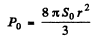or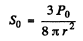Thus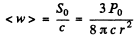(Poynting flux vector is the energy contained is a box of unit cross section and length c).

Q.221. An electric dipole whose modulus is constant and whose moment is equal to p rotates with constant angular velocity w about the axis drawn at right angles to the axis of the dipole and passing through its midpoint. Find the power radiated by such a dipole.

Ans. The rotating dispole has moments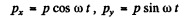Thus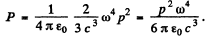Q.222. A free electron is located in the field of a plane electromagnetic wave. Neglecting the magnetic component of the wave disturbing its motion, find the ratio of the mean energy radiated by the oscillating electron per unit time to the mean value of the energy flow density of the incident wave.

Ans. If the electric field of the wave is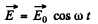then this induces a dipole moment whose second derivative is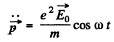Hence radiated mean power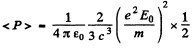On the other hand the mean Poynting flux of the incident radiation is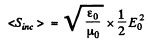Thus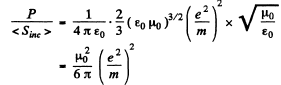Q.223. A plane electromagnetic wave with frequency ω falls upon an elastically bonded electron whose natural frequency equals ω0. Neglecting the damping of oscillations, find the ratio of the mean energy dissipated by the electron per unit time to the mean value of the energy flow density of the incident wave.

Ans. For the elastically bound electron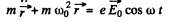This equation has the particular integral (i.e. neglecting the part which does not have the frequency of the impressed force)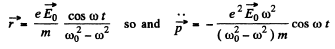Hence P = mean radiated power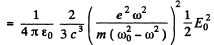The mean incident poynting flux is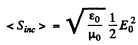Thus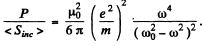Q.224. Assuming a particle to have the form of a ball and to absorb all incident light, find the radius of a particle for which its gravitational attraction to the Sun is counterbalanced by the force that light exerts on it. The power of light radiated by the Sun equals P = 4.1026  W, and the density of the particle is p = 1.0 g/cm3

Ans. Let r = radius of the ball R = distance betw een the ball & the Sun ( r « R ) .
M = mass of the Sun

y = gravitational constant

Then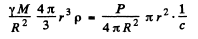( the factor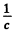converts the energy received on the right into momentum received. Then the right hand side is the momentum received per unit time and must equal tLe negative of the impressed force for equilibrium).

Thus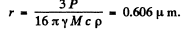The document Irodov Solutions: Electromagnetic Waves. Radiation- 2 - Notes | Study I. E. Irodov Solutions for Physics Class 11 & Class 12 - JEE is a part of the JEE Course I. E. Irodov Solutions for Physics Class 11 & Class 12.
All you need of JEE at this link: JEE

111 docs

## I. E. Irodov Solutions for Physics Class 11 & Class 12

111 docs

Track your progress, build streaks, highlight & save important lessons and more!

,

,

,

,

,

,

,

,

,

,

,

,

,

,

,

,

,

,

,

,

,

;